# Gann Angles Indicator for ThinkorSwim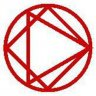#### Picard

##### New member
Here's code for Gann Angles originally coded by Mobius and modified for highs and lows by someone else. I posted this for those who already know how to use Gann's Angles. There are courses in Udemy.com, if you don't know already. Also look here: The Best Gann Fan Trading Strategy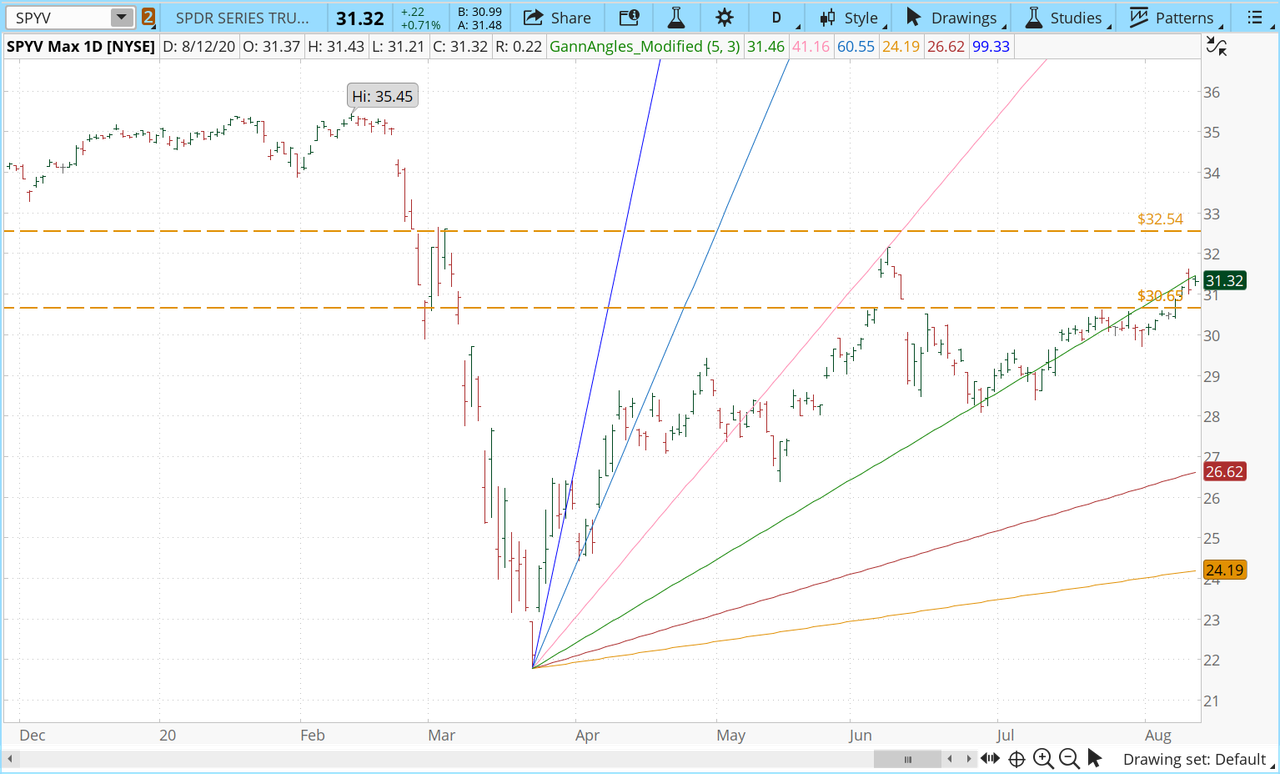Code:
``````# Gann Angles by Mobius
# Modified
# V02.03.03.2019
#hint: The primary Gann angles: 1X2, 1X1 and 2X1.  1X1 is moving one unit of price with one unit of time. Additional angles 1X8, 1X4, 4X1 and 8X1.
def Coefficient_1 = .5;
def Coefficient_2 = 1;
def Coefficient_3 = 2;
def Coefficient_4 = .125;
def Coefficient_5 = .25;
def Coefficient_6 = 4;
def bar = BarNumber();

input numMonths = 1;
input lineWeight = 3;

def numBars     = 21 * numMonths;
def barNum       = if IsNaN( close ) then Double.NaN else BarNumber();
def endBar      = HighestAll( barNum );
def startBar    = if endBar <= numBars then 1 else endBar - numBars;

def hData       = If( barNum < startBar, Double.NaN, high );
def lData       = If( barNum < startBar, Double.NaN, low );

def peak = HighestAll(hData);

def Apex = HighestAll(hData);
def Apex_Bar = if high == Apex
then bar
else Double.NaN;

then bar
else Double.NaN;

def FirstValue = if HighestAll(Apex_Bar) == FirstBar
then Apex
else FirstValue;

def LastValue = if HighestAll(Apex_Bar) == LastBar
then Apex
else LastValue;

# Gann Line Algorithm
def x = AbsValue(HighestAll(Apex_Bar) - HighestAll(Nadir_Bar));
def slope_1 = (AbsValue(Apex - Nadir) * Coefficient_1) / x;
def slope_2 = (AbsValue(Apex - Nadir) * Coefficient_2) / x;
def slope_3 = (AbsValue(Apex - Nadir) * Coefficient_3) / x;
def slope_4 = (AbsValue(Apex - Nadir) * Coefficient_4) / x;
def slope_5 = (AbsValue(Apex - Nadir) * Coefficient_5) / x;
def slope_6 = (AbsValue(Apex - Nadir) * Coefficient_6) / x;

plot G1 = if bar >= FirstBar and HighestAll(Apex_Bar) == FirstBar
then FirstValue - (slope_1 * (bar - FirstBar))
else if bar >= FirstBar and HighestAll(Nadir_Bar) == FirstBar
then FirstValue + (slope_1 * (bar - FirstBar))
else Double.NaN;
plot G2 = if bar >= FirstBar and HighestAll(Apex_Bar) == FirstBar
then FirstValue - (slope_2 * (bar - FirstBar))
else if bar >= FirstBar and HighestAll(Nadir_Bar) == FirstBar
then FirstValue + (slope_2 * (bar - FirstBar))
else Double.NaN;
plot G3 = if bar >= FirstBar and HighestAll(Apex_Bar) == FirstBar
then FirstValue - (slope_3 * (bar - FirstBar))
else if bar >= FirstBar and HighestAll(Nadir_Bar) == FirstBar
then FirstValue + (slope_3 * (bar - FirstBar))
else Double.NaN;
plot G4 = if bar >= FirstBar and HighestAll(Apex_Bar) == FirstBar
then FirstValue - (slope_4 * (bar - FirstBar))
else if bar >= FirstBar and HighestAll(Nadir_Bar) == FirstBar
then FirstValue + (slope_4 * (bar - FirstBar))
else Double.NaN;
plot G5 = if bar >= FirstBar and HighestAll(Apex_Bar) == FirstBar
then FirstValue - (slope_5 * (bar - FirstBar))
else if bar >= FirstBar and HighestAll(Nadir_Bar) == FirstBar
then FirstValue + (slope_5 * (bar - FirstBar))
else Double.NaN;
plot G6 = if bar >= FirstBar and HighestAll(Apex_Bar) == FirstBar
then FirstValue - (slope_6 * (bar - FirstBar))
else if bar >= FirstBar and HighestAll(Nadir_Bar) == FirstBar
then FirstValue + (slope_6 * (bar - FirstBar))
else Double.NaN;
# End Code Gann Angles``````

Last edited:#### TonXas

##### New member
VIP
Any way to modify this to draw a -45 degree angle from the HOD and a 45 degree angle from the LOD?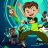Gann-OverExtended Indicator for ThinkorSwim Custom 2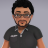Gann High Low Indicator for ThinkorSwim Indicators 19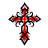Z-Score Upper Indicator for ThinkorSwim Indicators 4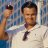Aroon Indicator Crossover Arrow? Questions 2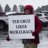Thinkscript for Moving Average from different Index/Indicator Questions 0